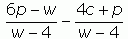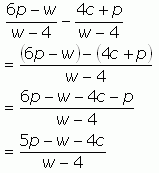SEARCH HOMEMath Central Quandaries & QueriesQuestion from pamela, a parent: Subtract:Hi Pamela.

The important thing is that when you are adding or subtracting rational expressions, you must make sure you first have a common denominator. In the question you sent us, there already is a common denominator of w - 4, so you can simply add the numerators and simplify.The numerator in the final expression doesn't factor, so this is in simplest form now.

Cheers,
Stephen La Rocque.Math Central is supported by the University of Regina and The Pacific Institute for the Mathematical Sciences.